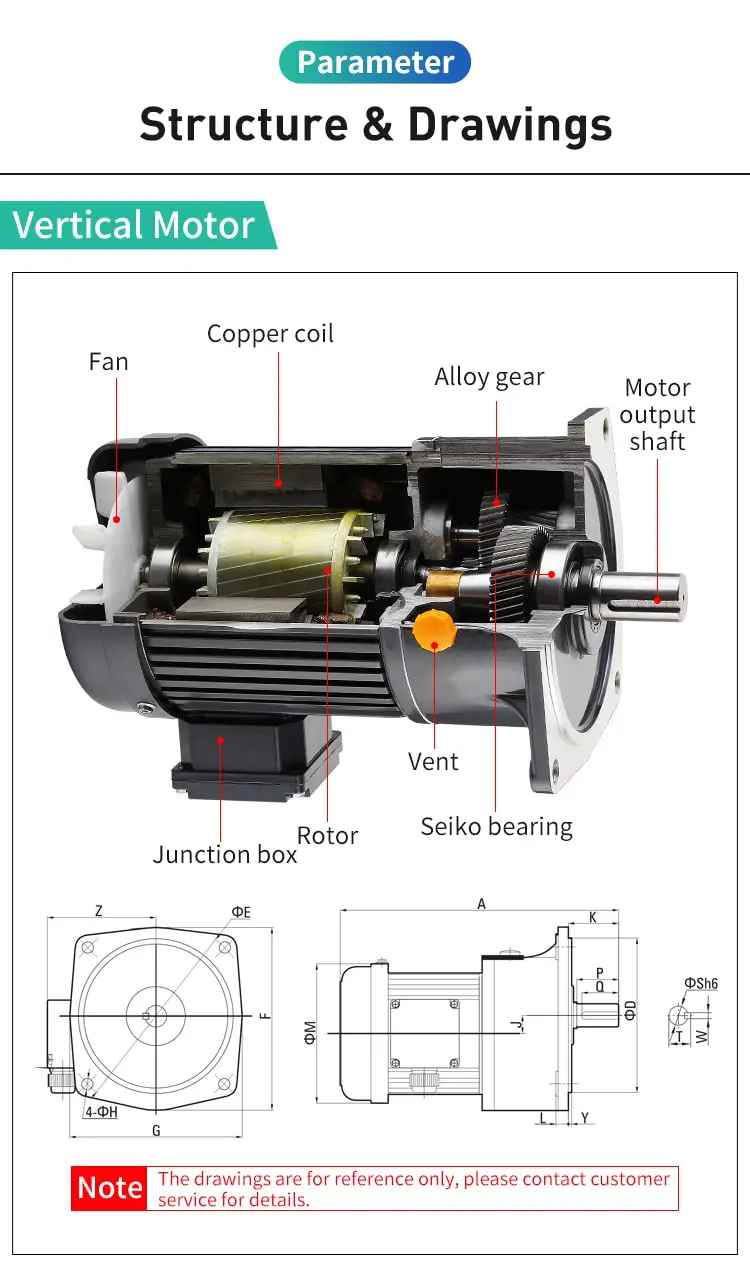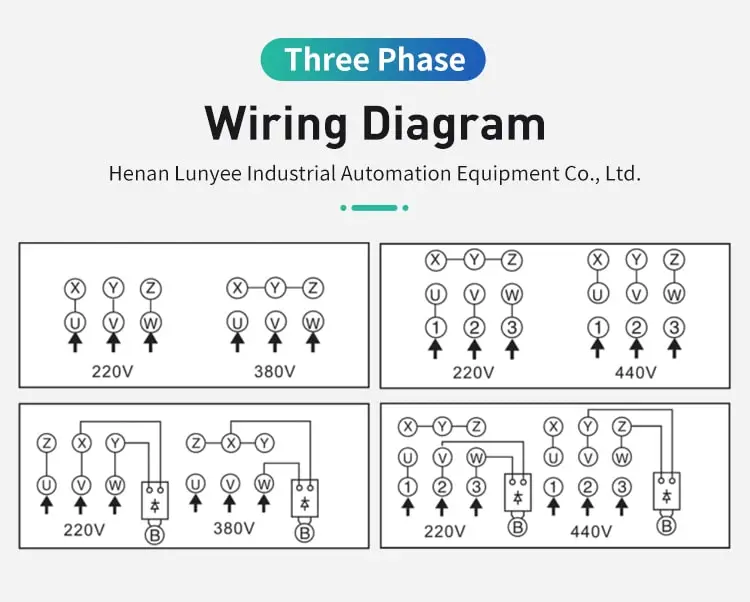By Jasmine 2023-02-24 17:13:21

#### How to calculate power factor of induction motor?

Working power and reactive power make up Apparent Power, which is called kVA, kilovolt-amperes. We determine apparent power using the formula, kVA2 = kV*A. Going one step further, Power Factor (PF) is the ratio of working power to apparent power, or the formula PF = kW / kVA.

The power factor of an induction motor can be calculated using the following formula:

Power Factor = (Real Power / Apparent Power) x 100%

To calculate the power factor of an induction motor, you will need to measure the real power and the apparent power of the motor. The real power of the motor is the actual power that is being used to do work, and it can be measured using a wattmeter or a power meter. The apparent power of the motor is the total power that is being supplied to the motor, and it can be measured using a voltmeter and an ammeter.

Once you have measured the real power and the apparent power of the induction motor , you can use the above formula to calculate the power factor of the motor. For example, if the real power of the motor is 1000 watts and the apparent power of the motor is 1200 volt-amperes, then the power factor of the motor would be calculated as follows:

Power Factor = (1000 watts / 1200 volt-amperes) x 100% = 83.3%

In this example, the power factor of the induction motor is 83.3%, which means that 83.3% of the power that is being supplied to the motor is being used to do useful work, and the remaining 16.7% is being wasted as heat or other forms of energy.

In summary, the power factor of an induction motor can be calculated using the formula: Power Factor = (Real Power / Apparent Power) x 100%. To calculate the power factor of an induction motor, you will need to measure the real power and the apparent power of the motor, and then use the above formula to calculate the power factor of the motor.